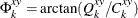# The SPECTRA Procedure

### OUT= Data Set

The OUT= data set contains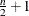observations, if n is even, or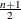observations, if n is odd, where n is the number of observations in the time series or the span of data being analyzed if missing values are present in the data. See the section Missing Values for details.

The variables in the new data set are named according to the following conventions. Each variable to be analyzed is associated with an index. The first variable listed in the VAR statement is indexed as 01, the second variable as 02, and so on. Output variables are named by combining indexes with prefixes. The prefix always identifies the nature of the new variable, and the indices identify the original variables from which the statistics were obtained.

Variables that contain spectral analysis results have names that consist of a prefix, an underscore, and the index of the variable analyzed. For example, the variable S_01 contains spectral density estimates for the first variable in the VAR statement. Variables that contain cross-spectral analysis results have names that consist of a prefix, an underscore, the index of the first variable, another underscore, and the index of the second variable. For example, the variable A_01_02 contains the amplitude of the cross-spectral density estimate for the first and second variables in the VAR statement.

Table 26.3 shows the formulas and naming conventions used for the variables in the OUT= data set. Let X be variable number nn in the VAR statement list and let Y be variable number mm in the VAR statement list. Table 26.3 shows the output variables that contain the results of the spectral and cross-spectral analysis of X and Y.

In Table 26.3 the following notation is used. Let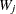be the vector of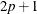smoothing weights given by the WEIGHTS statement, normalized to sum to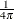. Note that the weights are either explicitly provided using the constant specification or are implicitly determined by the kernel specification in the WEIGHTS statement.

The subscript ofruns from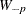to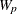, so that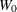is the middle weight in the list. Let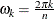, where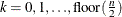.

Table 26.3: Variables Created by PROC SPECTRA

Variable

Description

FREQ

frequency in radians from 0 to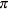(Note: Cycles per observation is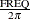.)

PERIOD

period or wavelength: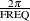(Note: PERIOD is missing for FREQ=0.)

COS_nn

cosine transform of X: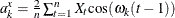SIN_nn

sine transform of X: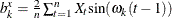P_nn

periodogram of X: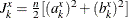S_nn

spectral density estimate of X: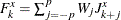(except across endpoints)

RP_nn _mm

real part of cross-periodogram X and Y: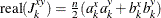IP_nn _mm

imaginary part of cross-periodogram of X and Y: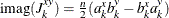CS_nn _mm

cospectrum estimate (real part of cross-spectrum) of X and Y: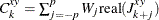(except across endpoints)

QS_nn _mm

quadrature spectrum estimate (imaginary part of cross-spectrum) of X and Y: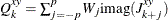(except across endpoints)

A_nn _mm

amplitude (modulus) of cross-spectrum of X and Y: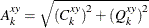K_nn _mm

coherency squared of X and Y: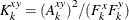PH_nn _mm

phase spectrum in radians of X and Y: